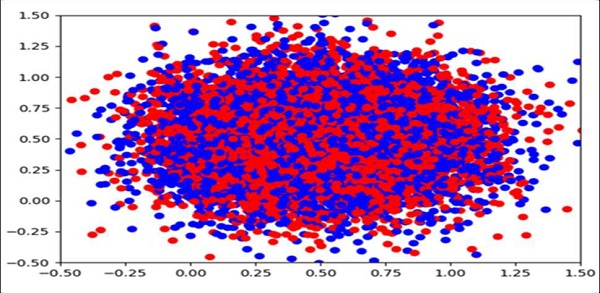# How can I make a scatter plot colored by density in Matplotlib?

We can create a dict for color and a value. If the same value comes up, we can use a scatter method and if the closer values have the same set of colors, that could make the plot color denser.

## Steps

• Create a new figure, or activate an existing figure.

• Add an ~.axes.Axes to the figure as part of a subplot arrangement.

• Get the x and y values using np.random.normal() method. Draw random samples from a normal (Gaussian) distribution.

• Make a color list with red and blue colors.

• To make it denser, we can store the same color with the same value.

• Plot scatter point, a scatter plot of *y* vs. *x* with varying marker size and/or color.

• Set the x-view limit.

• Set the y-view limit.

• To show the figure, use the plt.show() method.

## Example

import random
import numpy as np
import matplotlib.pyplot as plt

fig = plt.figure()

x = np.random.normal(0.5, 0.3, 10000)
y = np.random.normal(0.5, 0.3, 10000)

colors = ['red', 'blue']

color = dict()
for i in x:
if i not in color:
color[i] = colors[random.randint(1, 10) % len(colors)]

ax.scatter(x, y, c=[color.get(i) for i in x])

ax.set_xlim(-0.5, 1.5)
ax.set_ylim(-0.5, 1.5)

plt.show()

## Output